# §12.3赛道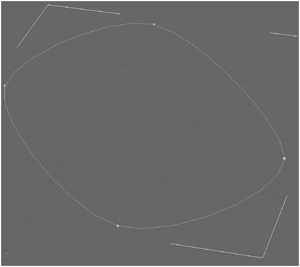## 单元测试

public static void TestRenderingTrack()

{

TrackLine testTrack = new TrackLine( new Vector3[] { new Vector3(20, -20, 0), new Vector3(20, 20, 0), new Vector3(-20, 20, 0), new Vector3(-20, -20, 0),

}

);

TestGame.Start(

delegate

{

ShowGroundGrid();

ShowTrackLines(testTrack);

ShowUpVectors(testTrack);

}

);

} // TestRenderingTrack()

ShowGroundGrid方法只是在xy平面上显示一些网格线以帮助你知道地面在哪。之后我在model类中写了这个方法，能够被重复使用。ShowTrackLines是最重要的方法，因为它显示了所有的线条和插值点，这些线条和插值点已在TrackLine类的构造函数中生成。最后ShowUpVectors方法告诉向量的向上方向供赛道每个点使用。没有向上向量，你将无法正确地生成道路的左右两侧。例如，在曲线赛道上应该倾斜、在循环赛道上你需要向上向量指向圆轨道圆心，而不只是向上。

ShowTrackLines辅助方法显示赛道的每个点，它们之间通过白色直线连接。当你执行TestRenderingTrack单元测试后就可以看到如图12-13的画面。

public static void ShowTrackLines(TrackLine track)

{

// Draw the line for each line part

for (int num = 0; num < track.points.Count; num++)

BaseGame.DrawLine( track.points[num].pos, track.points[(num + 1)%track.points.Count].pos, Color.White);

} // ShowTrackLines(track)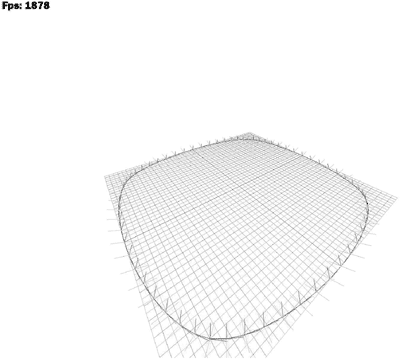public static void TestRenderingTrack()

{

TrackLine testTrack = new TrackLine( new Vector3[] { new Vector3(0, 0, 0), new Vector3(0, 7, 3), new Vector3(0, 10, 10), new Vector3(0, 7, 17), new Vector3(0, 0, 20), new Vector3(0, -7, 17), new Vector3(0, -10, 10), new Vector3(0, -7, 3),

}

); // [Rest stays the same]

} // TestRenderingTrack()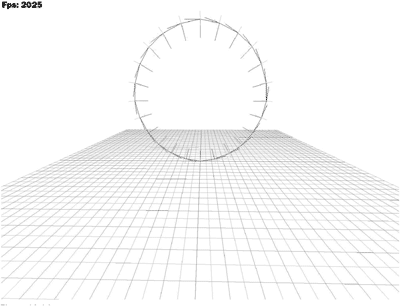## 插值样条

• 检查赛道上的每一点看看它是否在场景之上。如果没有，该点会被纠正，而且周围的点也会稍微上升少许以使赛道看起来更光滑。通过这种方式，你可以轻松地在Max中生成一个三维赛道，而当将赛道放置在场景之上时，就无需担心场景的实际高度。
• 圆轨道被简化为上下两个取样点。加载代码会自动检测这两个点并用完整循环的九个点取代它们，这样插入更多的点以产生非常光滑和正确的圆轨道。然后，所有赛道上的点通过Catmull-Rom插值方法被插值。你马上就会看到这种方法。
• 向上和切线向量会生成并插值好几次以使道路尽可能平滑。切线向量尤其不应该突然改变方向或翻转到另一边，这将使得在这条道路上开车变得非常困难。这个代码我花费了最长的时间才使之能工作正常。
• 然后，所有分析所有辅助类和对应赛道上的每一个点的道路宽度被储存，以便接下去使用，实际渲染发生在Track类，它是基于TrackLine类的。 道路纹理的纹理坐标也在这里生成，因为你将所有赛道点的信息存储在TrackVertex数组中，这样可以使接下去的渲染更容易。只有u纹理坐标是储存在这里的，而v纹理坐标在后来只是被设置为0或1，这取决于你是在道路的左边还是右边。
• 然后，分析隧道辅助类并生成隧道数据。这里的代码只是构建了一些新的点供以后使用。它们被用来在Track类中绘制带有隧道纹理的隧道盒。
• 最后所有的场景模型被添加。他们和赛道数据一起被保存为一个完整的关卡。附加的场景物体也在Track类中自动生成，例如，路边的路灯等。

// Generate all points with help of catmull rom splines

for (int num = 0; num < inputPoints.Length; num++)

{

// Get the 4 required points for the catmull rom spline

Vector3 p1 = inputPoints[num-1 < 0 ? inputPoints.Length-1 : num-1];

Vector3 p2 = inputPoints[num];

Vector3 p3 = inputPoints[(num + 1) % inputPoints.Length];

Vector3 p4 = inputPoints[(num + 2) % inputPoints.Length];

// Calculate number of iterations we use here based

// on the distance of the 2 points we generate new points from. float distance = Vector3.Distance(p2, p3);

int numberOfIterations = (int)(NumberOfIterationsPer100Meters * (distance / 100.0f));

if (numberOfIterations <= 0)

numberOfIterations = 1;

Vector3 lastPos = p1;

for (int iter = 0; iter < numberOfIterations; iter++)

{

TrackVertex newVertex = new TrackVertex( Vector3.CatmullRom(p1, p2, p3, p4, iter / (float)numberOfIterations));

} // for (iter)

} // for (num)

## 更复杂的赛道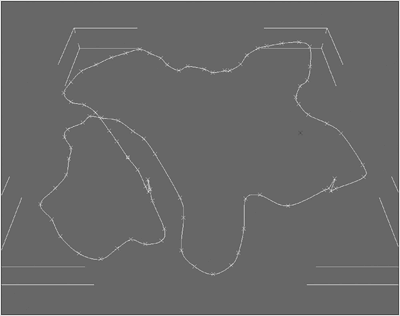## 导入赛道数据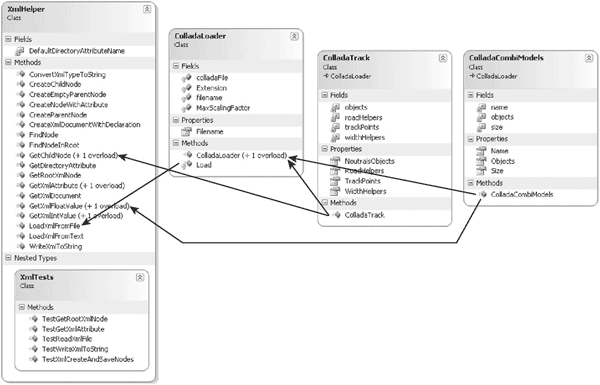{

// Get spline first (only use one line)

string splineId = XmlHelper.GetXmlAttribute(geometry, "id");

// Make sure this is a spline, everything else is not supported.

if (splineId.EndsWith("-spline") == false)

throw new Exception("The ColladaTrack file " + Filename + " does not have a spline geometry in it. Unable to load " + "track!");

// Get spline points XmlNode pointsArray = XmlHelper.GetChildNode(geometry, "float_array");

// Convert the points to a float array float[] pointsValues = StringHelper.ConvertStringToFloatArray( pointsArray.FirstChild.Value);

// Skip first and third of each input point (MAX tangent data)

trackPoints.Clear();

int pointNum = 0;

while (pointNum < pointsValues.Length) {

// Skip first point (first 3 floating point values) pointNum += 3;

// Take second vector trackPoints.Add(MaxScalingFactor * new Vector3( pointsValues[pointNum++], pointsValues[pointNum++], pointsValues[pointNum++]));

// And skip thrid

pointNum += 3;

}

// while (pointNum)

// Check if we can find translation or scaling values for our

// spline XmlNode

splineInstance = XmlHelper.GetChildNode( visualScene, "url", "#" + splineId);

XmlNode splineMatrixNode = XmlHelper.GetChildNode( splineInstance.ParentNode, "matrix");

if (splineMatrixNode != null)

throw new Exception("The ColladaTrack file " + Filename + " should not use baked matrices. Please export again " + "without baking matrices. Unable to load track!");

XmlNode splineTranslateNode = XmlHelper.GetChildNode( splineInstance.ParentNode, "translate");

XmlNode splineScaleNode = XmlHelper.GetChildNode( splineInstance.ParentNode, "scale");

Vector3 splineTranslate = Vector3.Zero;

if (splineTranslateNode != null)

{

float[] translateValues = StringHelper.ConvertStringToFloatArray( splineTranslateNode.FirstChild.Value);

splineTranslate = MaxScalingFactor * new Vector3( translateValues, translateValues, translateValues);

}

// if (splineTranslateNode)

Vector3 splineScale = new Vector3(1, 1, 1);

if (splineScaleNode != null)

{

float[] scaleValues = StringHelper.ConvertStringToFloatArray( splineScaleNode.FirstChild.Value);

splineScale = new Vector3( scaleValues, scaleValues, scaleValues);

}

// if (splineTranslateNode)

// Convert all points with our translation and scaling values

for (int num = 0; num < trackPoints.Count; num++)

{

trackPoints[num] = Vector3.Transform(trackPoints[num], Matrix.CreateScale(splineScale) * Matrix.CreateTranslation(splineTranslate));

}

// for (num)

## 从赛道数据生成顶点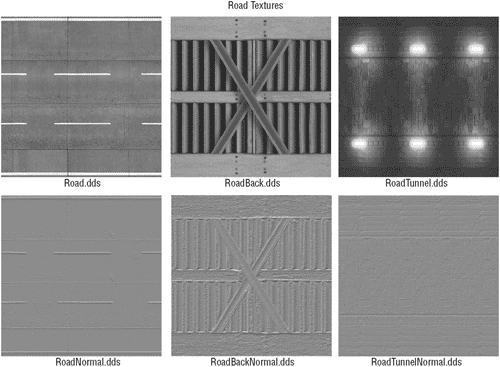TrackLine类处理所有这些纹理，有道路材质，道路水泥柱、护栏、检查站等，它是基于，它从Track类继承而来的。

Landscape类用来绘制赛道和所有场景物体以及场景本身，最后才能使汽车在道路上行驶。你还需要物理学处理在赛道上的运动、与护栏的碰撞，这在下一章会说到。

public static void TestRenderTrack()

{

Track track = null;

TestGame.Start( delegate

{ track = new Track("TrackBeginner", null);

},

delegate

{

ShowUpVectors(track); track.Render();

}

);

} // TestRenderingTrack()

public void Render()

{

// We use tangent vertices for everything here

BaseGame.Device.VertexDeclaration = TangentVertex.VertexDeclaration;

// Restore the world matrix

BaseGame.WorldMatrix = Matrix.Identity;

delegate {

BaseGame.Device.DrawIndexedPrimitives( PrimitiveType.TriangleList, 0, 0, points.Count * 5, 0, (points.Count - 1) * 8);

}

); // [etc. Render rest of road materials]

} // Render()

private void GenerateVerticesAndObjects(Landscape landscape)

{

// Each road segment gets 5 points:

// left, left middle, middle, right middle, right.

// The reason for this is that we would have bad triangle errors if the

// road gets wider and wider. This happens because we need to render

// quads, but we can only render triangles, which often have different

// orientations, which makes the road very bumpy. This still happens

// with 8 polygons instead of 2, but it is much better this way.

// Another trick is to not do so many iterations in TrackLine, which

// causes this problem. Better to have a not so round track, but at

// least the road up/down itself is smooth.

// The last point is duplicated (see TrackLine) because we have 2 sets

// of texture coordinates for it (begin block, end block).

// So for the index buffer we only use points.Count-1

blocks. roadVertices = new TangentVertex[points.Count * 5];

// Current texture coordinate for the roadway (in direction of

// movement)

for (int num = 0; num < points.Count; num++)

{ // Get vertices with the help of the properties in the TrackVertex

// class. For the road itself we only need vertices for the left

// and right side, which are vertex number 0 and 1.

roadVertices[num * 5 + 0] = points[num].RightTangentVertex;

roadVertices[num * 5 + 1] = points[num].MiddleRightTangentVertex;

roadVertices[num * 5 + 2] = points[num].MiddleTangentVertex;

roadVertices[num * 5 + 3] = points[num].MiddleLeftTangentVertex;

roadVertices[num * 5 + 4] = points[num].LeftTangentVertex;

} // for (num)

// Also calculate all indices, we have 8 polygons for each segment

// with 3 vertices each. We have 1 segment less than points because

// the last point is duplicated (different tex coords).

int[] indices = new int[(points.Count - 1) * 8 * 3];

int vertexIndex = 0;

for (int num = 0; num < points.Count - 1; num++)

{ // We only use 3 vertices (and the next 3 vertices),

// but we have to construct all 24 indices for our 4 polygons.

for (int sideNum = 0; sideNum < 4; sideNum++)

{

// Each side needs 2 polygons.

// 1. Polygon

indices[num * 24 + 6 * sideNum + 0] = vertexIndex + sideNum;

indices[num * 24 + 6 * sideNum + 1] = vertexIndex + 5 + 1 + sideNum;

indices[num * 24 + 6 * sideNum + 2] = vertexIndex + 5 + sideNum;

// 2. Polygon

indices[num * 24 + 6 * sideNum + 3] = vertexIndex + 5 + 1 + sideNum;

indices[num * 24 + 6 * sideNum + 4] = vertexIndex + sideNum;

indices[num * 24 + 6 * sideNum + 5] = vertexIndex + 1 + sideNum;

} // for (num)

// Go to the next 5 vertices

vertexIndex += 5;

} // for (num)

// Set road back index buffer

roadIb = new IndexBuffer( BaseGame.Device, typeof(int), indices.Length, ResourceUsage.WriteOnly, ResourceManagementMode.Automatic);

#endregion

// [Then the rest of the road back, tunnel, etc. vertices are

// generated here and all the landscape objects, checkpoints, palms,

// etc. are generated at the end of this method]

} // GenerateVerticesAndObjects(landscape)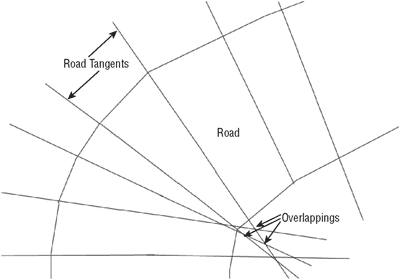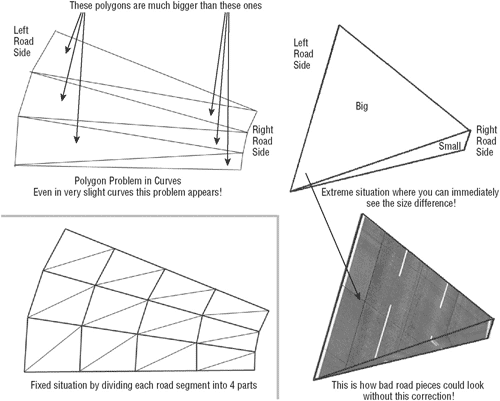## 最后结果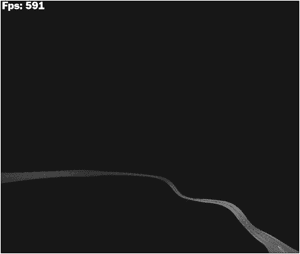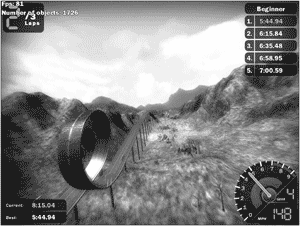2006 - 2023，推荐分辨率1024*768以上，推荐浏览器Chrome、Edge等现代浏览器，截止2021年12月5日的访问次数：1872万9823 站长邮箱